Python Date Time Tutorial with Examples

View more Tutorials: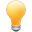Follow us on our fanpages to receive notifications every time there are new articles.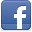Facebook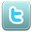Twitter

1- Overview

Python provides you with 4 modules related to date and time.
 Module Description time time is a module that only includes functions, and constants related to date and time, there are several classes written in C/C ++ defined on this module. For example, the struct_time class. datetime datetime is a module which is designed with object-oriented programming to work with date and time in Python. It defines several classes that represent date and time. calendar The calendar is a module that provides functions, and several classes related to Calendar, which support generating images of the calendar as text, html, .... locale This module contains functions that are used for formatting, or parsing date and time based on locale.
datetime module:
datetime is a module, designed base on object oriented to work with date and time in Python. It has several classes that represent date and time.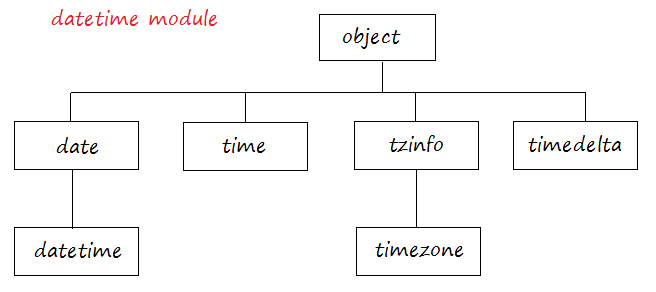Class Discription datetime.date A date object represents a date, excluding time, according to the Gregorian calendar. datetime.datetime A datetime object represents a date and time, according to the Gregorian calendar. datetime.time A time object represents time, excluding date. datetime.tzinfo A base abstract class for time zone information objects. datetime.timezone A direct child class of the tzinfo class, in UTC (Coordinated Universal Time). datetime.timedelta A timedelta object represents a duration, the difference between two dates or times.
calendar module: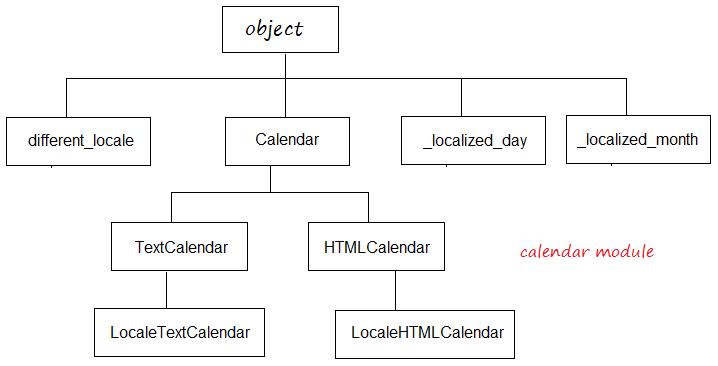The calendar is a module that provides functions, and several classes related to Calendar, which support generating images of the calendar as text, html, ....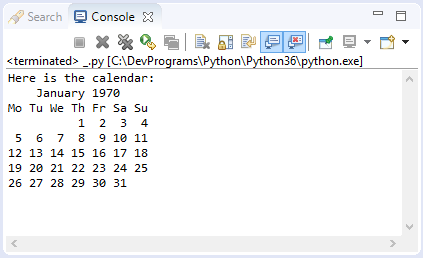2- The concept of Ticks

In computer science, 12 am on January 1, 1970 is a special time, it is used to start counting time. This special moment is called epoch (Computer Age).

In Python, the time between the present moment and the special time above is expressed in seconds. That time period is called Ticks.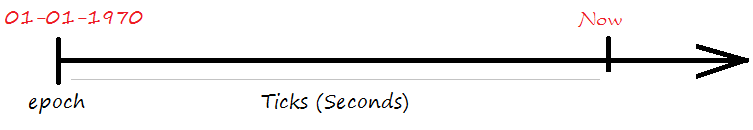The time() function in the time module returns the number of seconds from 12 am on January 1, 1970 to the present.
ticketExample.py

# Import time module.
import time;

# Number of seconds since 12:00am, January 1, 1970
ticks = time.time()
print ("Number of ticks since 12:00am, January 1, 1970: ", ticks)
Output:

Number of ticks since 12:00am, January 1, 1970: 1492244686.7766237

3- time module

Time is a module that only includes functions, and constants related to date and time, there are several classes written in C/C ++ defined on this module. For example, the struct_time class.
On module time, time is represented by Ticks or struct_time. It has functions that format Ticks or struct_time into strings, and vice versa parse a string into Ticks or struct_time.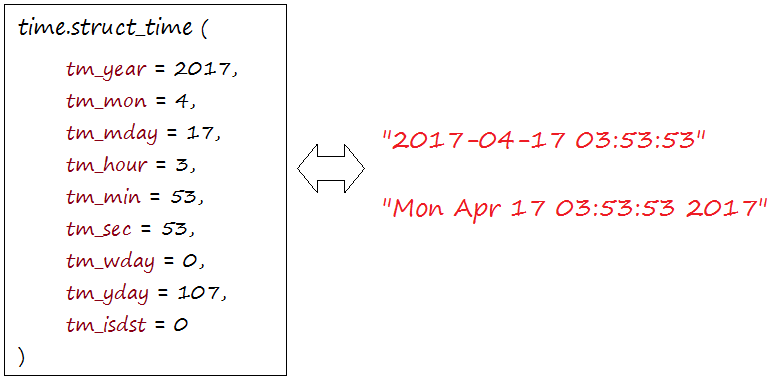Index Attribute Values
0 tm_year (for example, 1993)
1 tm_mon range [1, 12]
2 tm_mday range [1, 31]
3 tm_hour range [0, 23]
4 tm_min range [0, 59]
5 tm_sec range [0, 61]; See more below
6 tm_wday range [0, 6], Monday is 0
7 tm_yday range [1, 366]
8 tm_isdst 0, 1 or -1; see below
N/A tm_zone abbreviation of timezone name
N/A tm_gmtoff offset east of UTC in seconds
tm_sec
The range of second really is 0 to 61; this accounts for leap seconds and the (very rare) double leap seconds.
The functions of time call functions written in C language. Here is a list of common functions, in order to detail it, you can refer to document on official website of Python.
Ticks ==> struct_time
 Function Discription time.gmtime([secs]) Convert the time in seconds from the spoch time to a struct_time in UTC, where the dst flag is 0. If the secs parameter is not supplied or None, it will have the default value returned by the time() function. time.localtime([secs]) The same as gmtime() function, but is converted into local time. And the dst flag has a value of 1.
The gmtime([secs]) and localtime([secs]) functions return the struct_time type.
time_gmtimeExample.py

import time

# 1 seconds after epoch.
# This function return a struct: struct_time
ts = time.gmtime(1)
print ("1 seconds after epoch: ")
print (ts)
print ("\n")

# Now, same as time.gmtime( time.time() )
# This function return a struct: struct_time
ts = time.gmtime()
print ("struct_time for current time: ")
print (ts)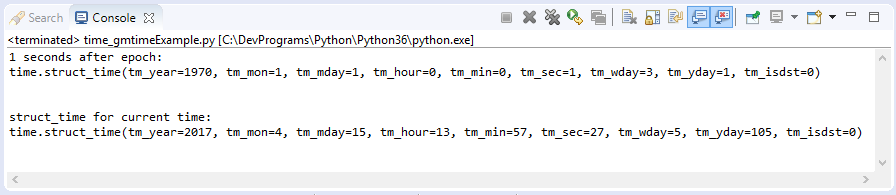struct_time ==> Ticks
You can convert a struct_time or a Tuple representing the time into Ticks (the number of seconds is calculated from the time of the spoch).
time_mktime_example.py

import time

a_struct_time = time.localtime()
print ("Current time as struct_time: ");
print (a_struct_time)
# Convert struct_time or Tuple to Ticks.
ticks = time.mktime(a_struct_time)
print ("Ticks: ", ticks)

# A Tuple with 9 elements.
aTupleTime = ( 2017, 4, 15, 13, 5, 34, 0, 0, 0)
print ("\n")
print ("A Tuple represents time: ")
print (aTupleTime)

# Convert struct_time or Tuple to Ticks.
ticks = time.mktime(aTupleTime)
print ("Ticks: ", ticks)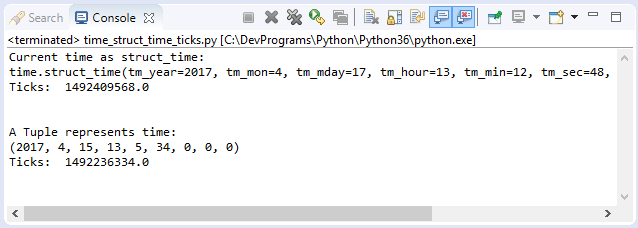struct_time, Ticks ==> string
 Function Description time.asctime([struct_t]) Convert a tuple or struct_time representing a time as returned by gmtime() or localtime() to a string of the following form: 'Sun Jun 20 23:21:05 1993'. If t is not provided, the current time as returned by localtime() is used. Locale information is not used by asctime(). time.ctime([secs]) Convert a time expressed in seconds since the epoch to a string representing local time. If secs is not provided or None, the current time as returned by time() is used. ctime(secs) is equivalent to asctime(localtime(secs)). Locale information is not used by ctime().
time_asctime_ctime_example.py

import time

# A Tuple with 9 elements.
# (Year, month, day, hour, minute, second, wday, yday, isdst)
a_tuple_time = (2017, 4, 15 , 22 , 1, 29, 0, 0, 0)
a_timeAsString = time.asctime(a_tuple_time)
print ("time.asctime(a_tuple_time): ", a_timeAsString)

a_struct_time = time.localtime()
print ("a_struct_time: ", a_struct_time)

a_timeAsString = time.asctime(a_struct_time)
print ("time.asctime(a_struct_time): ", a_timeAsString)

# The number of seconds since 1-1-1970 12am to current time.
ticks = time.time()
a_timeAsString = time.ctime(ticks)
print ("time.ctime(ticks): ", a_timeAsString)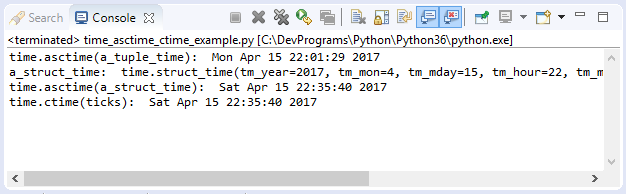Parse and format
Module time provides some functions used to parse a string into time. And vice versa, format the time into a string.
 Function Description time.strptime(string[, format] ) Parse a string representing a time according to a format. The return value is a struct_time as returned by gmtime() or localtime(). time.strftime(format [, t] ) Convert a tuple or struct_time representing a time as returned by gmtime() or localtime() to a string as specified by the format argument. If t is not provided, the current time as returned by localtime() is used. format must be a string. ValueError is raised if any field in 't' is outside of the allowed range.
An example of parsing a string into a struct_time.
time_strptime_example.py

import time
# A string representing time.
aStringTime = "22-12-2007 23:30:59"

a_struct_time = time.strptime(aStringTime, "%d-%m-%Y %H:%M:%S")
print ("a_struct_time:")
print (a_struct_time)
See more time module:

4- datetime module

Datetime is a module which is designed with object-oriented programming to work with date and time in Python. It defines several classes that represent date and time.Class Discription datetime.date A date object represents a date, excluding time, according to the Gregorian calendar. datetime.datetime A datetime object represents a date and time, according to the Gregorian calendar. datetime.time A time object represents time, excluding date. datetime.tzinfo A base abstract class for time zone information objects. datetime.timezone A direct child class of the tzinfo class, in UTC (Coordinated Universal Time). datetime.timedelta A timedelta object represents a duration, the difference between two dates or times.

5- datetime.timedelta

Timedelta is a class located in the datetime module, which describes a time period. As the difference between 2 time periods.
The timedelta class has seven attributes, all of which have a default value of 0.
 Attribute Attribute Range days -999999999 : 999999999 seconds 0 : 86399 microseconds 1 seconds = 1000,000 microseconds 0 : 999999 milliseconds 1 seconds = 1000 milliseconds minutes hours weeks
Operators:
Operation Example
t1 = t2 + t3 t2 = (hours = 10, seconds= 2)
t3 = (hours = 1, minutes = 3)
--> t1 = (hours= 11, minutes = 3, seconds = 2)
t1 = t2 - t3 t2 = (hours = 10, seconds= 2)
t3 = (hours = 1, minutes = 3)
--> t1 = (hours= 8, minutes = 57, seconds = 2)
t1 = t2 * i
t1 = i * t2
t2 = (hours = 10, seconds= 2)
i = 3
--> t1 = (days =1, hours = 6, seconds= 6)

t1 = t2 t2 = (hours = 25, seconds= 2)
--> t1 = (days: 1, hours: 1, seconds: 2)
+t1 return t1
-t1 t1 = (hours = 10, seconds= 2)
--> -t1 = (days = -1, hours = 13, minutes = 59, seconds= 58)
abs(t) Absolute value, equivalent to + t when t.days> = 0, and is -t when t.days <0.
t = (hours= -25, minutes = 3)
--> t = (days =  -2, hours = 23, minutes = 3)
--> abs(t) = (days =  1, hours = 0, minutes = 57)
str(t) Returns the string in the form [D day [s],] [H] H: MM: SS [.UUUUUU], D can get negative value.
repr(t) Returns a string in the form datetime.timedelta (D [, S [, U]]), D can get negative value

6- datetime.date

Datetime.date is a class, its object represents date, excluding time information
Constructor
** constructor **

# MINYEAR <= year <= MAXYEAR
# 1 <= month <= 12
# 1 <= day <= number of days in the given month and year
date (year, month, day)
The constructor of the date class can raise a ValueError if the values passed in are invalid (out of range).
Constants:
 Constants Description date.min The earliest representable date, date(MINYEAR, 1, 1). date.max The latest representable date, date(MAXYEAR, 12, 31). date.resolution The smallest possible difference between non-equal date objects, timedelta(days=1).
Operators
Operation Description
date2 = date1 + timedelta Add a duration, timedelta
date2 = date1 - timedelta Subtract a duration, timedelta
timedelta = date1 - date2 Subtract two date object.
date1 < date2 Compare two date objects.
Methods:
 Method Description date.replace(year=self.year, month=self.month, day=self.day) Return a date with the same value, except for the value replaced by the parameter. For example: d == date(2002, 12, 31), d.replace(day=26) == date(2002, 12, 26). date.timetuple() Return a time.struct_time such as returned by time.localtime(). The hours, minutes and seconds are 0, and the DST flag is -1. d.timetuple() is equivalent to time.struct_time((d.year, d.month, d.day, 0, 0, 0, d.weekday(), yday, -1)), where yday = d.toordinal() - date(d.year, 1, 1).toordinal() + 1 is the day number within the current year starting with 1 for January 1st. date.toordinal() Return the proleptic Gregorian ordinal of the date, where January 1 of year 1 has ordinal 1. For any date object d, date.fromordinal(d.toordinal()) == d. date.weekday() Return the day of the week as an integer, where Monday is 0 and Sunday is 6. For example, date(2002, 12, 4).weekday() == 2, a Wednesday. See also isoweekday(). date.isoweekday() Return the day of the week as an integer (According to ISO standard), where Monday is 1 and Sunday is 7. For example, date(2002, 12, 4).isoweekday() == 3, a Wednesday. See also weekday(), isocalendar(). date.isocalendar() Return a 3-tuple, (ISO year, ISO week number, ISO weekday). date.isoformat() Return a string representing the date in ISO 8601 format, ‘YYYY-MM-DD’. For example, date(2002, 12, 4).isoformat() == '2002-12-04'. date.__str__() For a date d, str(d) is equivalent to d.isoformat(). date.ctime() Return a string representing the date, for example date(2002, 12, 4).ctime() == 'Wed Dec 4 00:00:00 2002'. d.ctime() is equivalent to time.ctime(time.mktime(d.timetuple())). The native C ctime() function conforms to the C standard when it run on flatform. date.strftime(format) Return a string representing the date, In the format given by the parameter. Format codes referring to hours, minutes or seconds will see 0 values. See more strftime() and strptime() function of time module. date.__format__(format) Same as date.strftime().

View more Tutorials:

Maybe you are interested

These are online courses outside the o7planning website that we introduced, which may include free or discounted courses.

•Python 3 For Beginner - Object-Oriented Programming
•Complete Python Web Course: Build 8 Python Web Apps
•Master Computer Vision™ OpenCV4 in Python with Deep Learning
•Learning ECMAScript 6: Moving to the New JavaScript
•SwiftUI Quick Start Guide with iOS 13 and Xcode 11
•Learn C# From Scratch with Real Applications
•Learn iPython: The Full Python IDE
•Python GUI : From A-to-Z With 2 Final Projects
•Learning iPython Notebook
•Python Fundamentals
•Python MySql From Scratch
•Python Programming Full Course (Basics,OOP,Modules,PyQt)
•Learning Path: Python GUI Projects
•Learning Path: Python: Design and Architect Python Apps
•Creating Reports with SQL Server 2012 Reporting Services
•Python 3000: Tactical SQL Quick-Start
•Python BeautifulSoup: Extract Web Data Beautifully
•Python 3000: Tactical File I/O
•Web Programming with Python
•Master Java Web Services and RESTful API with Spring Boot
•New to Python Automation..?Try Step by Step Python 4 Testers
•Learning Path: Python Web Development
•Learn Programming in Python With the Power of Animation
•Python 2000: Beyond The Basics
•Distributed configuration with Spring Cloud Config